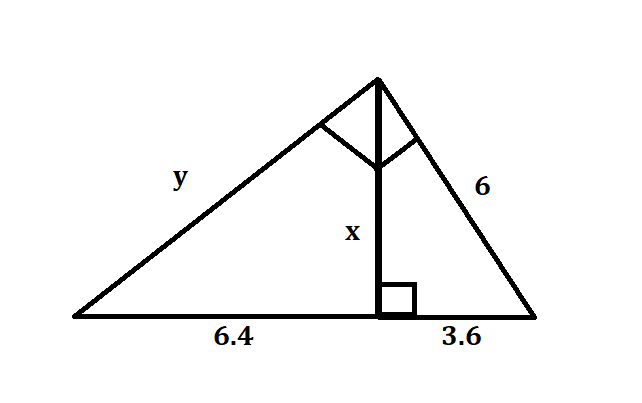Ask question

# Find the solution sin Y = cos ( Y + 20° )# Find the solution sin Y = cos ( Y + 20° )

Question
Right triangles and trigonometryasked 2021-03-07
Find the solution $$\displaystyle{\sin{{Y}}}={\cos{{\left({Y}+{20}°\right)}}}$$

## Answers (1)2021-03-08
In a right triangle the two acute angles add up to 90°. This means that the cosine of one angle is the sine of the other. So Y+Y+20=90, 2Y=70, Y=35°. There are other solutions but both the angles will not be acute.

### Relevant Questionsasked 2021-03-12
Aidan knows that the observation deck on the Vancouver Lookout is 130 m above the ground. He measures the angle between the ground and his line of sight to the observation deck as $$\displaystyle{77}^{\circ}$$. How far is Aidan from the base of the Lookout to the nearest metre?asked 2021-03-25
Find a general solution to $$\displaystyle{y}{''}+{4}{y}'+{3.75}{y}={109}{\cos{{5}}}{x}$$
To solve this, the first thing I did was find the general solutionto the homogeneous equivalent, and got
$$\displaystyle{c}_{{1}}{e}^{{-{5}\frac{{x}}{{2}}}}+{c}_{{2}}{e}^{{{3}\frac{{x}}{{2}}}}$$
Then i used the form $$\displaystyle{K}{\cos{{\left({w}{x}\right)}}}+{M}{\sin{{\left({w}{x}\right)}}}$$ and got $$\displaystyle-{2.72}{\cos{{\left({5}{x}\right)}}}+{2.56}{\sin{{\left({5}{x}\right)}}}$$ as a solution of the nonhomogeneous ODEasked 2020-12-27
Given theta is an acute angle such that sin theta = $$\displaystyle\frac{{5}}{{13}}$$ find the value of tan (theta - pi\4)asked 2020-11-26
Match each of the trigonometric expressions below with the equivalent non-trigonometric function from the following list. Enter the appropriate letter(A,B, C, D or E)in each blank
A . $$\displaystyle{\tan{{\left({\arcsin{{\left({\frac{{{x}}}{{{8}}}}\right)}}}\right)}}}$$
B . $$\displaystyle{\cos{{\left({a}{r}{\sin{{\left({\frac{{{x}}}{{{8}}}}\right)}}}\right)}}}$$
C. $$\displaystyle{\left({\frac{{{1}}}{{{2}}}}\right)}{\sin{{\left({2}{\arcsin{{\left({\frac{{{x}}}{{{8}}}}\right)}}}\right)}}}$$
D. $$\displaystyle{\sin{{\left({\arctan{{\left({\frac{{{x}}}{{{8}}}}\right)}}}\right)}}}$$
E. $$\displaystyle{\cos{{\left({\arctan{{\left({\frac{{{x}}}{{{8}}}}\right)}}}\right)}}}$$asked 2020-11-24
Find the exact value of y.asked 2020-10-26
Solve the equation
$$\displaystyle{\sin{{\left({x}°-{20}°\right)}}}={\cos{{42}}}°$$ for x, where 0 < x < 90asked 2021-01-13
The drawing shows a uniform electric field that points in the negative y direction; the magnitude of the field is 5300 N/C.Determine the electric potential difference (a) VB - VA between points A and B, (b) VC - VB between points B and C, and (c) VA - VB between points C and A.
A-C is 10.0cm, b-c is 8.0 cm, a-b is 6.0 cm. They are all in a right triangle shape. With angle b having the 90 degree angle, and electric potential is pointing down. This is problem 56 in 7th edition.asked 2020-11-16
Find the values of the variables in each right triangle.asked 2021-02-09
Use similar triangles to find the distance across the river.asked 2021-03-02
The lengths of the diagonals of a rectangle are represented by 8x + 3 feet and 4x + 7 feet. Find the length of each diagonal.
...